Скачать презентацию 7 Investment Analysis and Portfolio Management First Canadian

ed4c661f0d794f5cc3b4407b2e385316.ppt

• Количество слайдов: 67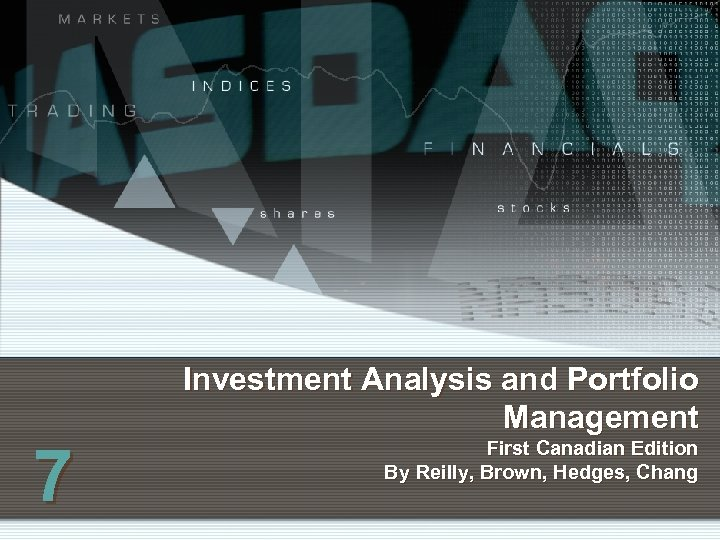7 Investment Analysis and Portfolio Management First Canadian Edition By Reilly, Brown, Hedges, Chang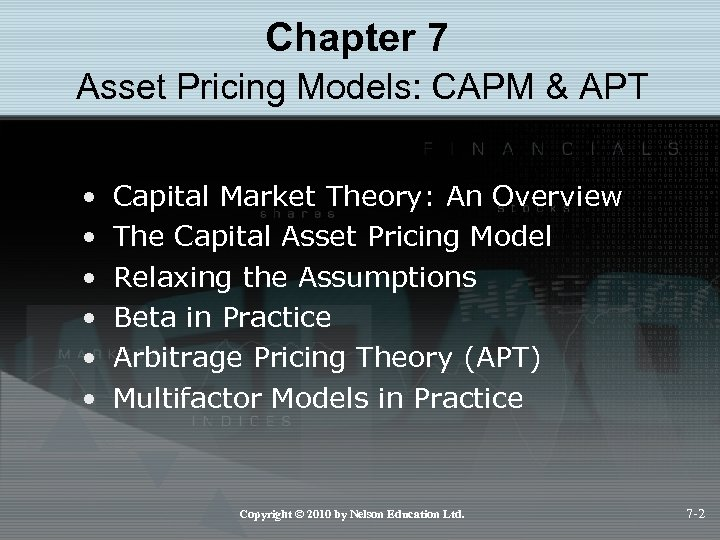Chapter 7 Asset Pricing Models: CAPM & APT • • • Capital Market Theory: An Overview The Capital Asset Pricing Model Relaxing the Assumptions Beta in Practice Arbitrage Pricing Theory (APT) Multifactor Models in Practice Copyright © 2010 by Nelson Education Ltd. 7 -2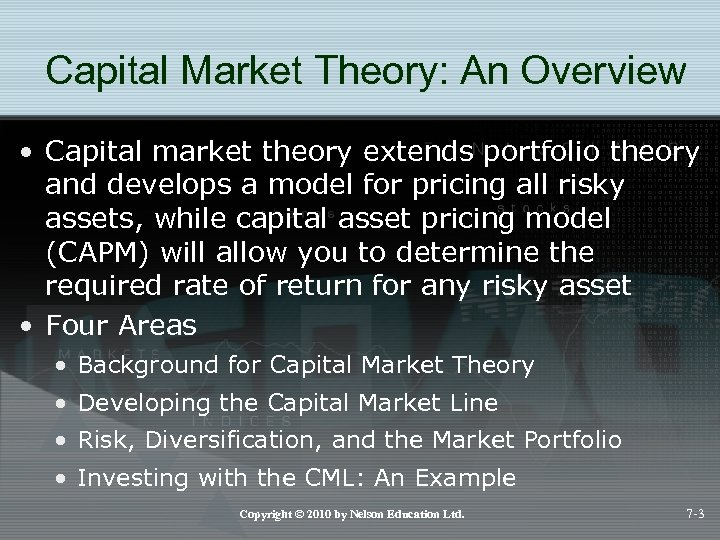Capital Market Theory: An Overview • Capital market theory extends portfolio theory and develops a model for pricing all risky assets, while capital asset pricing model (CAPM) will allow you to determine the required rate of return for any risky asset • Four Areas • Background for Capital Market Theory • Developing the Capital Market Line • Risk, Diversification, and the Market Portfolio • Investing with the CML: An Example Copyright © 2010 by Nelson Education Ltd. 7 -3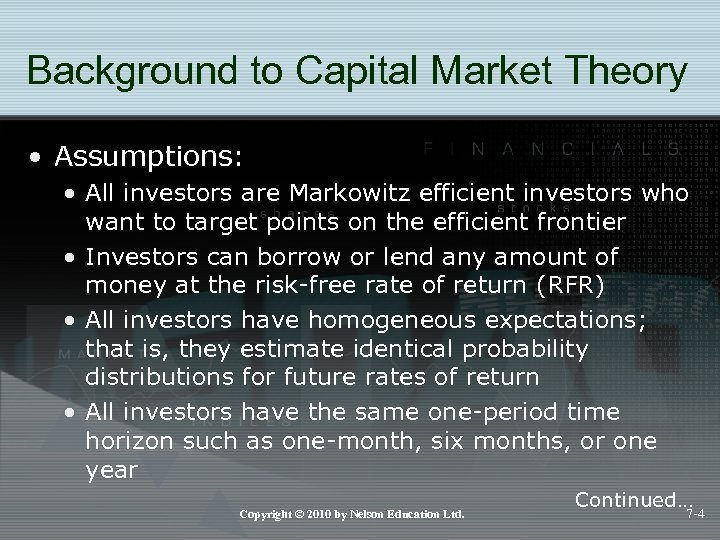Background to Capital Market Theory • Assumptions: • All investors are Markowitz efficient investors who want to target points on the efficient frontier • Investors can borrow or lend any amount of money at the risk-free rate of return (RFR) • All investors have homogeneous expectations; that is, they estimate identical probability distributions for future rates of return • All investors have the same one-period time horizon such as one-month, six months, or one year Copyright © 2010 by Nelson Education Ltd. Continued… 7 -4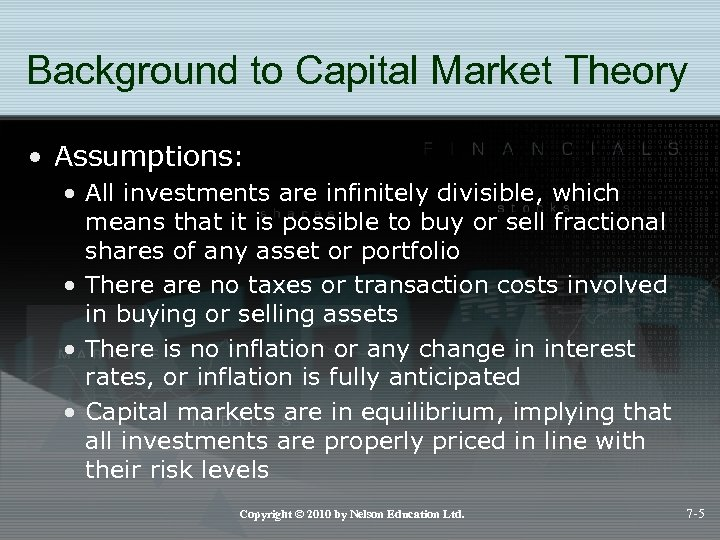Background to Capital Market Theory • Assumptions: • All investments are infinitely divisible, which means that it is possible to buy or sell fractional shares of any asset or portfolio • There are no taxes or transaction costs involved in buying or selling assets • There is no inflation or any change in interest rates, or inflation is fully anticipated • Capital markets are in equilibrium, implying that all investments are properly priced in line with their risk levels Copyright © 2010 by Nelson Education Ltd. 7 -5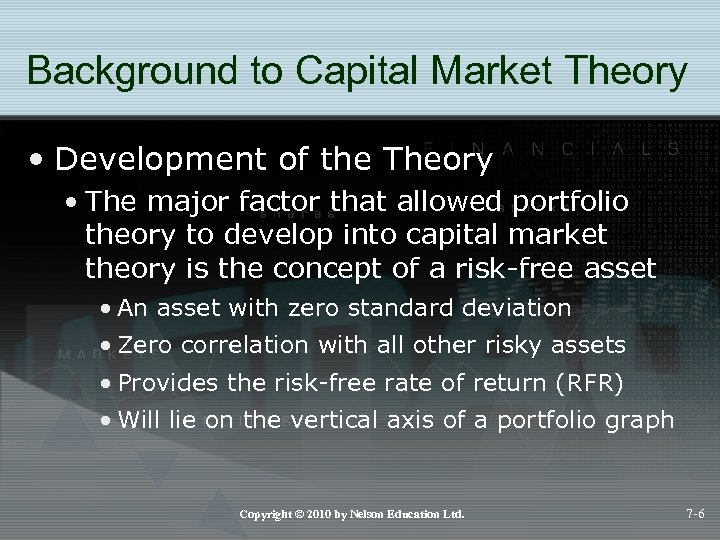Background to Capital Market Theory • Development of the Theory • The major factor that allowed portfolio theory to develop into capital market theory is the concept of a risk-free asset • An asset with zero standard deviation • Zero correlation with all other risky assets • Provides the risk-free rate of return (RFR) • Will lie on the vertical axis of a portfolio graph Copyright © 2010 by Nelson Education Ltd. 7 -6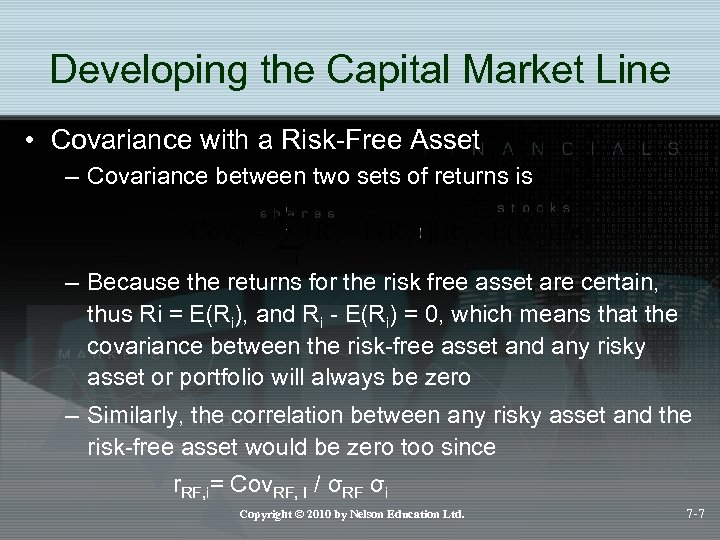Developing the Capital Market Line • Covariance with a Risk-Free Asset – Covariance between two sets of returns is – Because the returns for the risk free asset are certain, thus Ri = E(Ri), and Ri - E(Ri) = 0, which means that the covariance between the risk-free asset and any risky asset or portfolio will always be zero – Similarly, the correlation between any risky asset and the risk-free asset would be zero too since r. RF, i= Cov. RF, I / σRF σi Copyright © 2010 by Nelson Education Ltd. 7 -7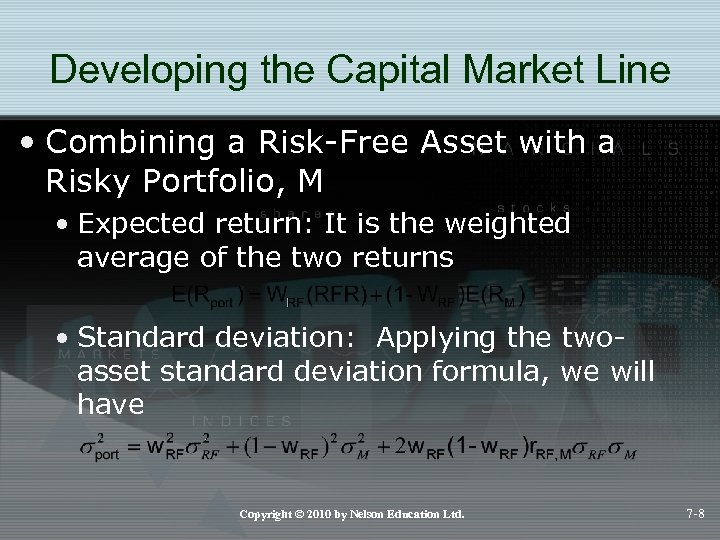Developing the Capital Market Line • Combining a Risk-Free Asset with a Risky Portfolio, M • Expected return: It is the weighted average of the two returns • Standard deviation: Applying the twoasset standard deviation formula, we will have Copyright © 2010 by Nelson Education Ltd. 7 -8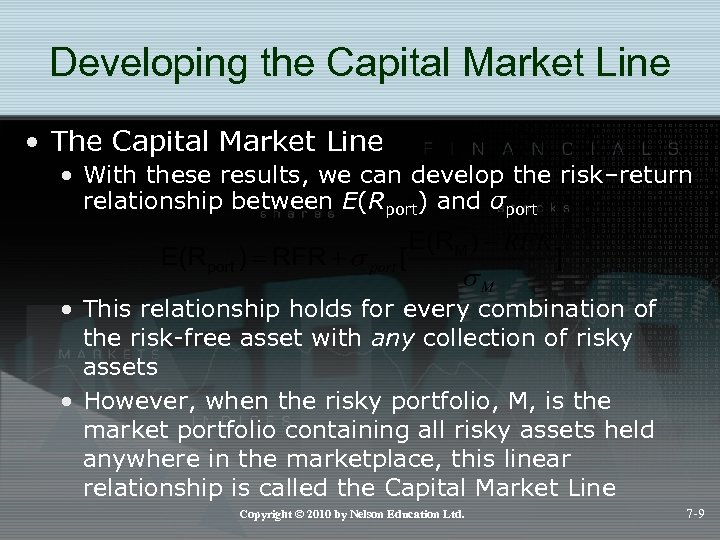Developing the Capital Market Line • The Capital Market Line • With these results, we can develop the risk–return relationship between E(Rport) and σport • This relationship holds for every combination of the risk-free asset with any collection of risky assets • However, when the risky portfolio, M, is the market portfolio containing all risky assets held anywhere in the marketplace, this linear relationship is called the Capital Market Line Copyright © 2010 by Nelson Education Ltd. 7 -9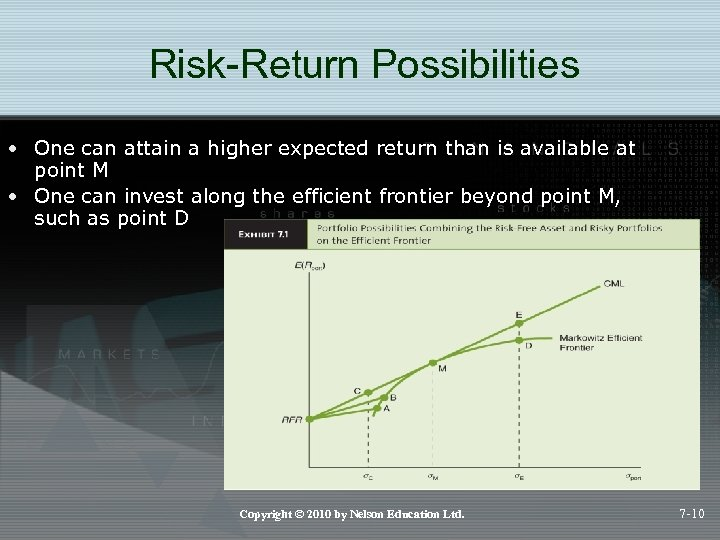Risk-Return Possibilities • One can attain a higher expected return than is available at point M • One can invest along the efficient frontier beyond point M, such as point D Copyright © 2010 by Nelson Education Ltd. 7 -10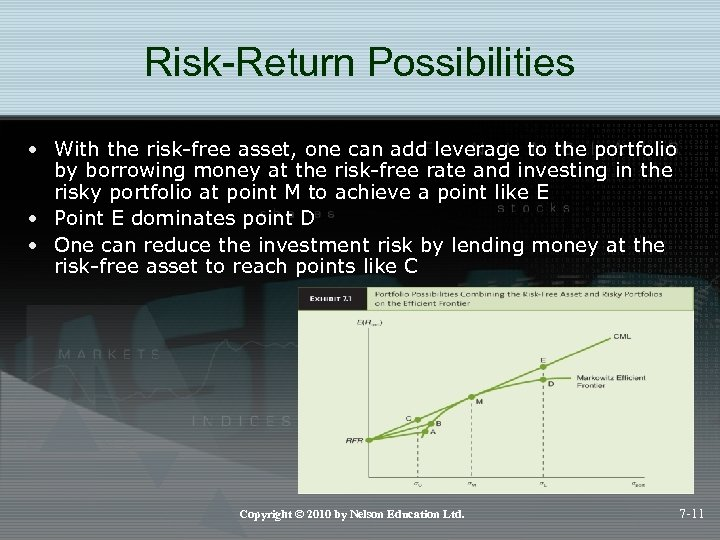Risk-Return Possibilities • With the risk-free asset, one can add leverage to the portfolio by borrowing money at the risk-free rate and investing in the risky portfolio at point M to achieve a point like E • Point E dominates point D • One can reduce the investment risk by lending money at the risk-free asset to reach points like C Copyright © 2010 by Nelson Education Ltd. 7 -11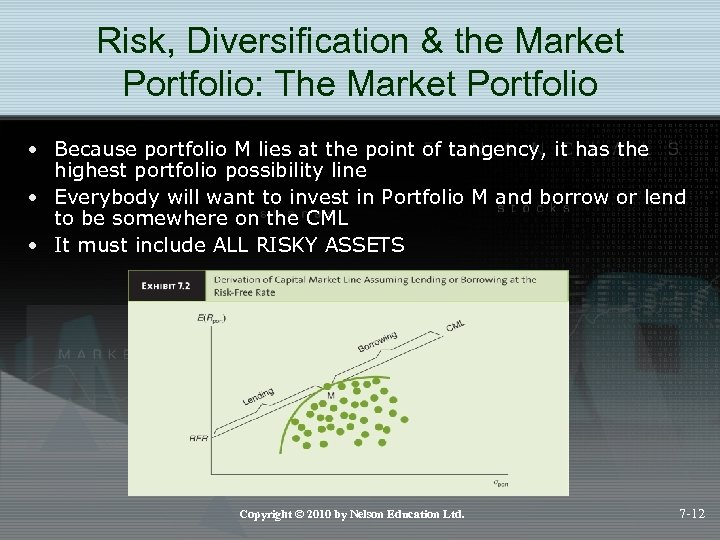Risk, Diversification & the Market Portfolio: The Market Portfolio • Because portfolio M lies at the point of tangency, it has the highest portfolio possibility line • Everybody will want to invest in Portfolio M and borrow or lend to be somewhere on the CML • It must include ALL RISKY ASSETS Copyright © 2010 by Nelson Education Ltd. 7 -12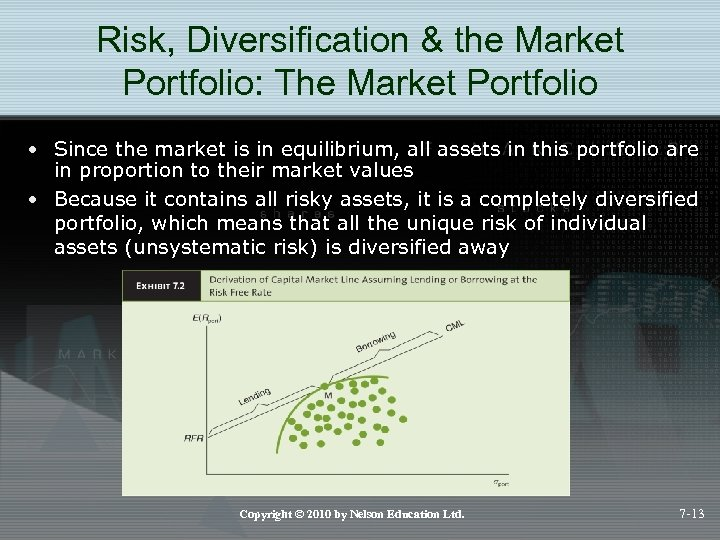Risk, Diversification & the Market Portfolio: The Market Portfolio • Since the market is in equilibrium, all assets in this portfolio are in proportion to their market values • Because it contains all risky assets, it is a completely diversified portfolio, which means that all the unique risk of individual assets (unsystematic risk) is diversified away Copyright © 2010 by Nelson Education Ltd. 7 -13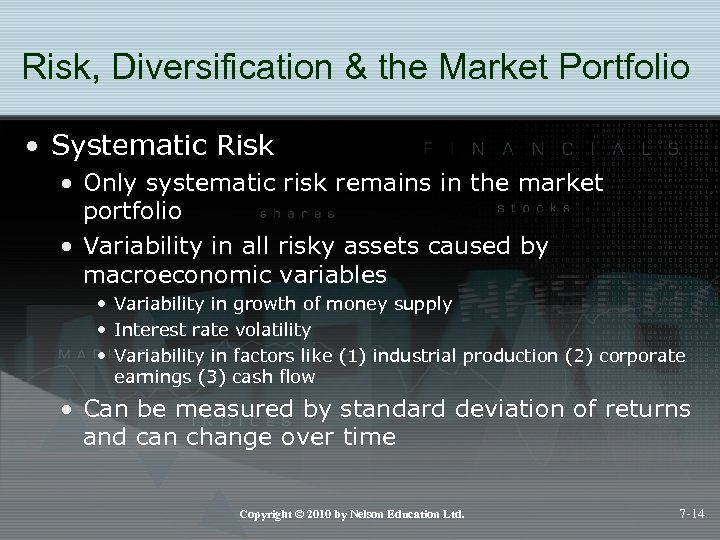Risk, Diversification & the Market Portfolio • Systematic Risk • Only systematic risk remains in the market portfolio • Variability in all risky assets caused by macroeconomic variables • Variability in growth of money supply • Interest rate volatility • Variability in factors like (1) industrial production (2) corporate earnings (3) cash flow • Can be measured by standard deviation of returns and can change over time Copyright © 2010 by Nelson Education Ltd. 7 -14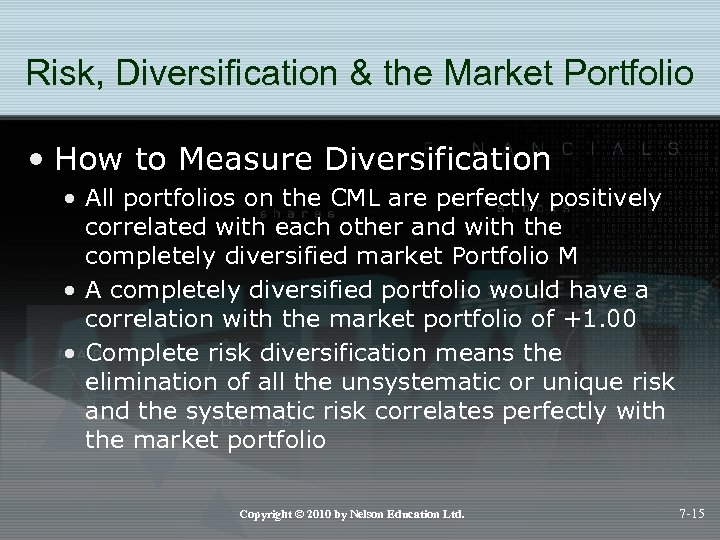Risk, Diversification & the Market Portfolio • How to Measure Diversification • All portfolios on the CML are perfectly positively correlated with each other and with the completely diversified market Portfolio M • A completely diversified portfolio would have a correlation with the market portfolio of +1. 00 • Complete risk diversification means the elimination of all the unsystematic or unique risk and the systematic risk correlates perfectly with the market portfolio Copyright © 2010 by Nelson Education Ltd. 7 -15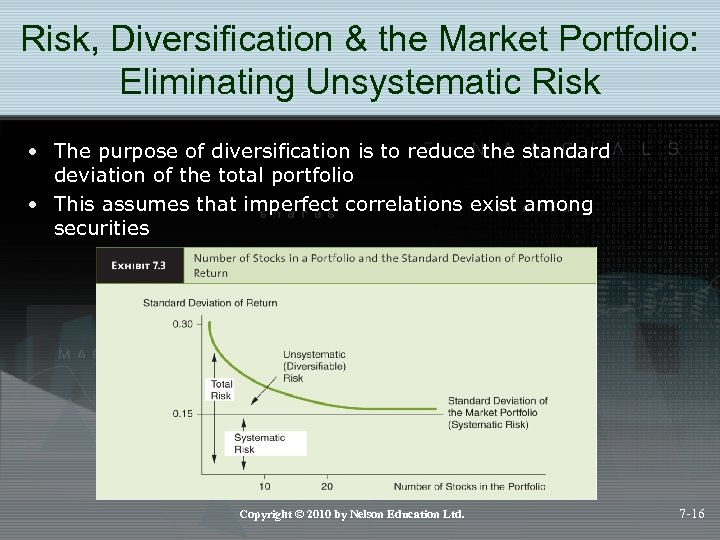Risk, Diversification & the Market Portfolio: Eliminating Unsystematic Risk • The purpose of diversification is to reduce the standard deviation of the total portfolio • This assumes that imperfect correlations exist among securities Copyright © 2010 by Nelson Education Ltd. 7 -16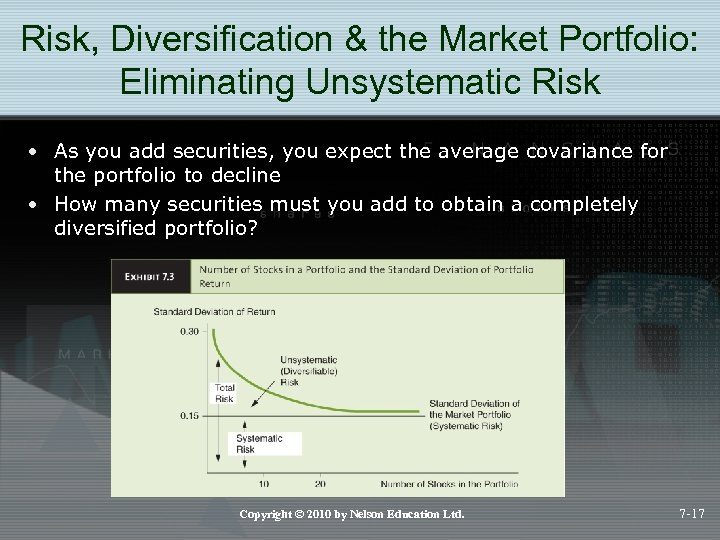Risk, Diversification & the Market Portfolio: Eliminating Unsystematic Risk • As you add securities, you expect the average covariance for the portfolio to decline • How many securities must you add to obtain a completely diversified portfolio? Copyright © 2010 by Nelson Education Ltd. 7 -17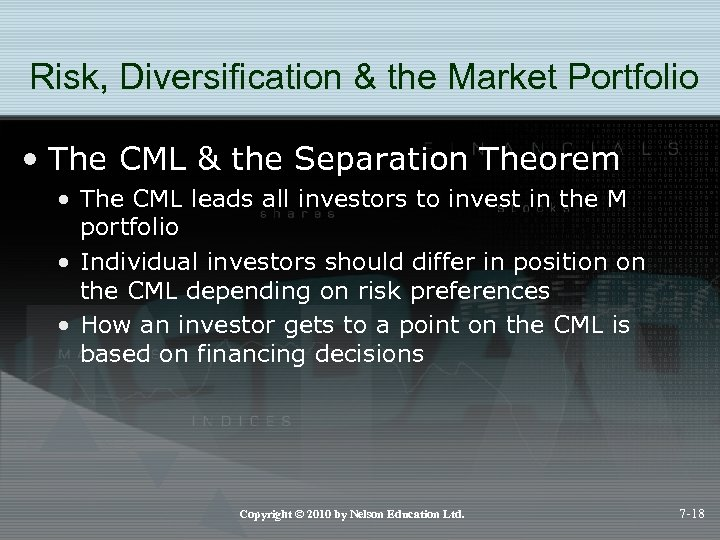Risk, Diversification & the Market Portfolio • The CML & the Separation Theorem • The CML leads all investors to invest in the M portfolio • Individual investors should differ in position on the CML depending on risk preferences • How an investor gets to a point on the CML is based on financing decisions Copyright © 2010 by Nelson Education Ltd. 7 -18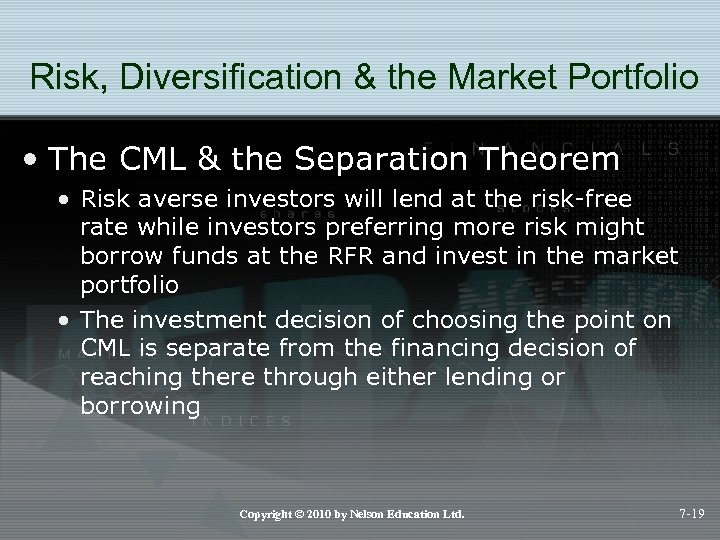Risk, Diversification & the Market Portfolio • The CML & the Separation Theorem • Risk averse investors will lend at the risk-free rate while investors preferring more risk might borrow funds at the RFR and invest in the market portfolio • The investment decision of choosing the point on CML is separate from the financing decision of reaching there through either lending or borrowing Copyright © 2010 by Nelson Education Ltd. 7 -19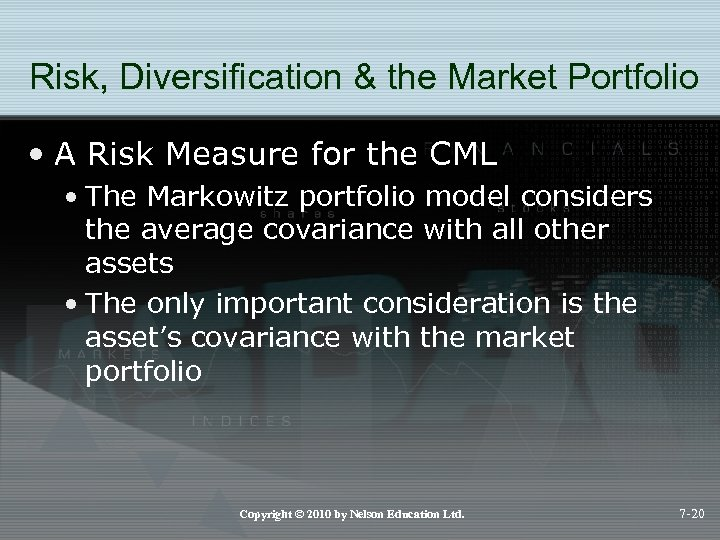Risk, Diversification & the Market Portfolio • A Risk Measure for the CML • The Markowitz portfolio model considers the average covariance with all other assets • The only important consideration is the asset’s covariance with the market portfolio Copyright © 2010 by Nelson Education Ltd. 7 -20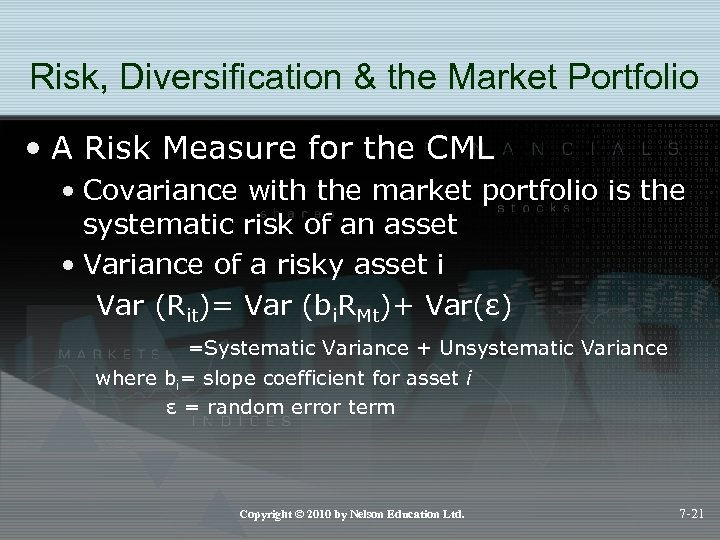Risk, Diversification & the Market Portfolio • A Risk Measure for the CML • Covariance with the market portfolio is the systematic risk of an asset • Variance of a risky asset i Var (Rit)= Var (bi. RMt)+ Var(ε) =Systematic Variance + Unsystematic Variance where bi= slope coefficient for asset i ε = random error term Copyright © 2010 by Nelson Education Ltd. 7 -21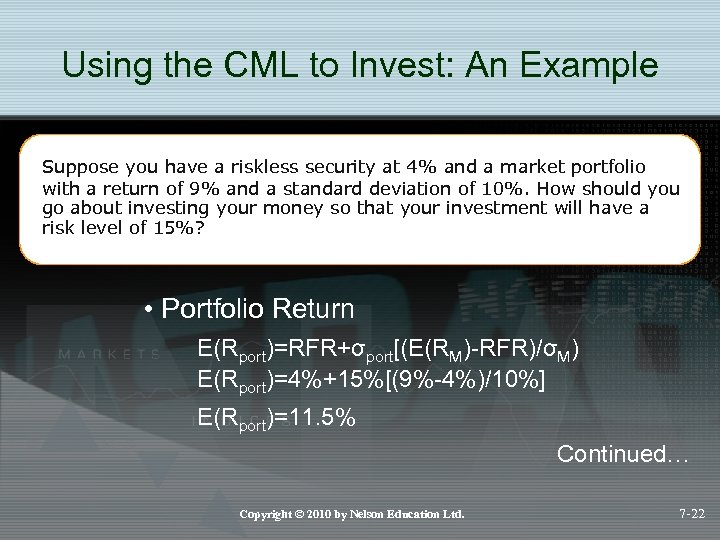Using the CML to Invest: An Example Suppose you have a riskless security at 4% and a market portfolio with a return of 9% and a standard deviation of 10%. How should you go about investing your money so that your investment will have a risk level of 15%? • Portfolio Return E(Rport)=RFR+σport[(E(RM)-RFR)/σM) E(Rport)=4%+15%[(9%-4%)/10%] E(Rport)=11. 5% Continued… Copyright © 2010 by Nelson Education Ltd. 7 -22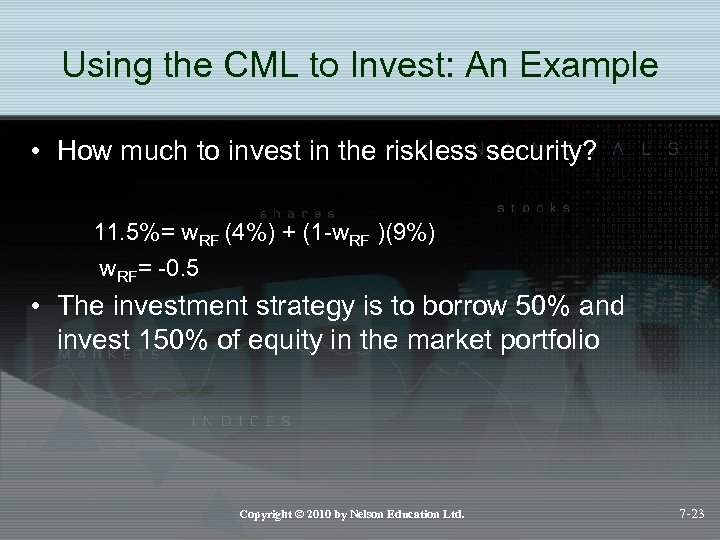Using the CML to Invest: An Example • How much to invest in the riskless security? 11. 5%= w. RF (4%) + (1 -w. RF )(9%) w. RF= -0. 5 • The investment strategy is to borrow 50% and invest 150% of equity in the market portfolio Copyright © 2010 by Nelson Education Ltd. 7 -23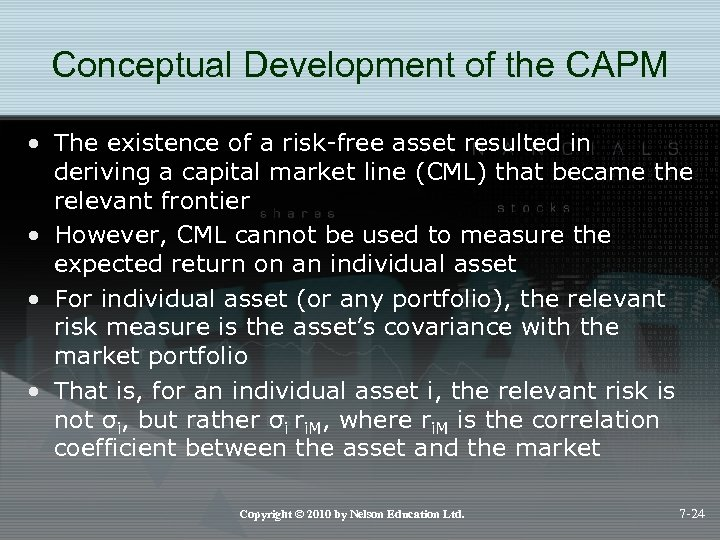Conceptual Development of the CAPM • The existence of a risk-free asset resulted in deriving a capital market line (CML) that became the relevant frontier • However, CML cannot be used to measure the expected return on an individual asset • For individual asset (or any portfolio), the relevant risk measure is the asset’s covariance with the market portfolio • That is, for an individual asset i, the relevant risk is not σi, but rather σi ri. M, where ri. M is the correlation coefficient between the asset and the market Copyright © 2010 by Nelson Education Ltd. 7 -24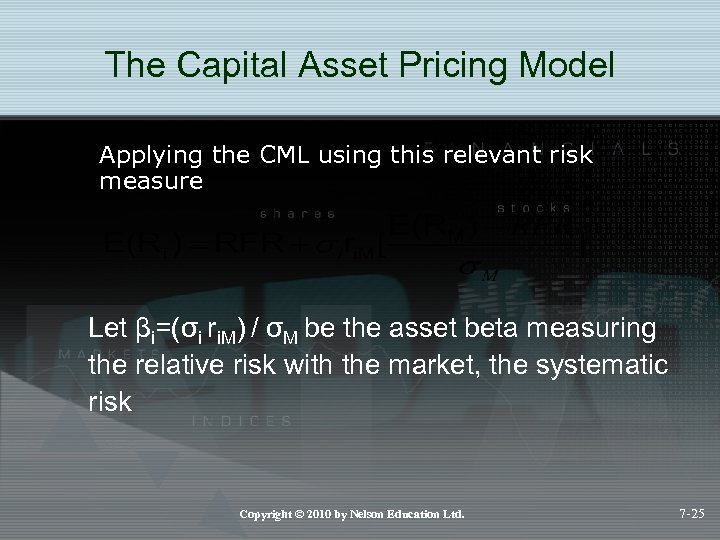The Capital Asset Pricing Model Applying the CML using this relevant risk measure Let βi=(σi ri. M) / σM be the asset beta measuring the relative risk with the market, the systematic risk Copyright © 2010 by Nelson Education Ltd. 7 -25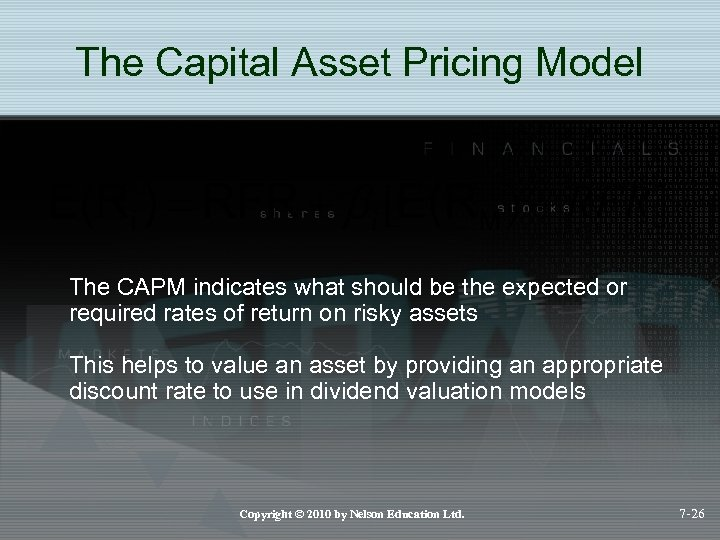The Capital Asset Pricing Model The CAPM indicates what should be the expected or required rates of return on risky assets This helps to value an asset by providing an appropriate discount rate to use in dividend valuation models Copyright © 2010 by Nelson Education Ltd. 7 -26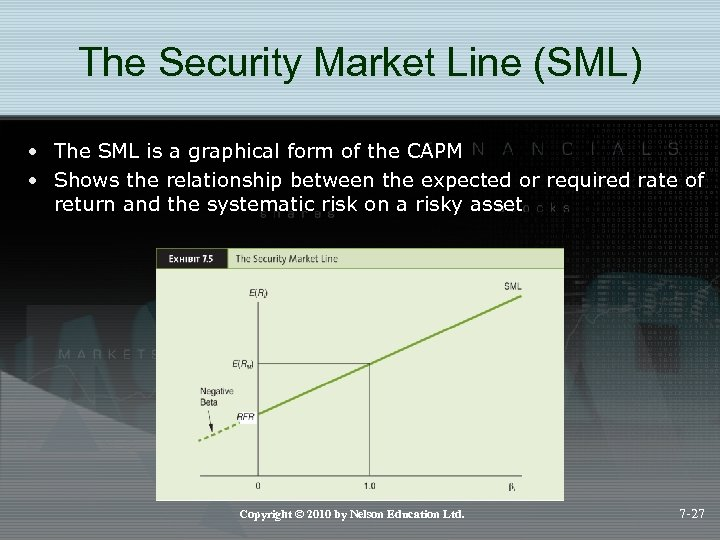The Security Market Line (SML) • The SML is a graphical form of the CAPM • Shows the relationship between the expected or required rate of return and the systematic risk on a risky asset Copyright © 2010 by Nelson Education Ltd. 7 -27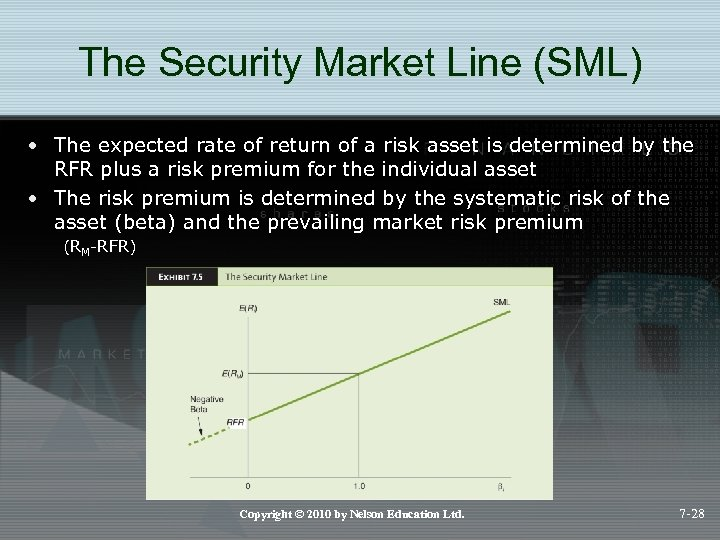The Security Market Line (SML) • The expected rate of return of a risk asset is determined by the RFR plus a risk premium for the individual asset • The risk premium is determined by the systematic risk of the asset (beta) and the prevailing market risk premium (RM-RFR) Copyright © 2010 by Nelson Education Ltd. 7 -28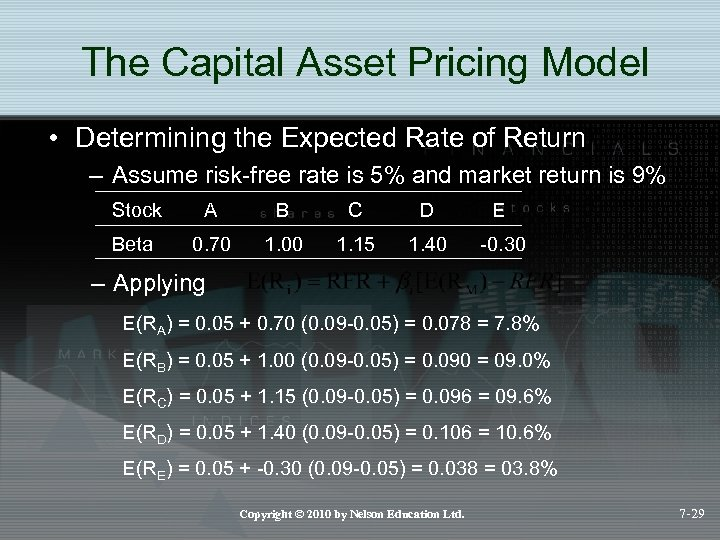The Capital Asset Pricing Model • Determining the Expected Rate of Return – Assume risk-free rate is 5% and market return is 9% Stock A B C D E Beta 0. 70 1. 00 1. 15 1. 40 -0. 30 – Applying E(RA) = 0. 05 + 0. 70 (0. 09 -0. 05) = 0. 078 = 7. 8% E(RB) = 0. 05 + 1. 00 (0. 09 -0. 05) = 0. 090 = 09. 0% E(RC) = 0. 05 + 1. 15 (0. 09 -0. 05) = 0. 096 = 09. 6% E(RD) = 0. 05 + 1. 40 (0. 09 -0. 05) = 0. 106 = 10. 6% E(RE) = 0. 05 + -0. 30 (0. 09 -0. 05) = 0. 038 = 03. 8% Copyright © 2010 by Nelson Education Ltd. 7 -29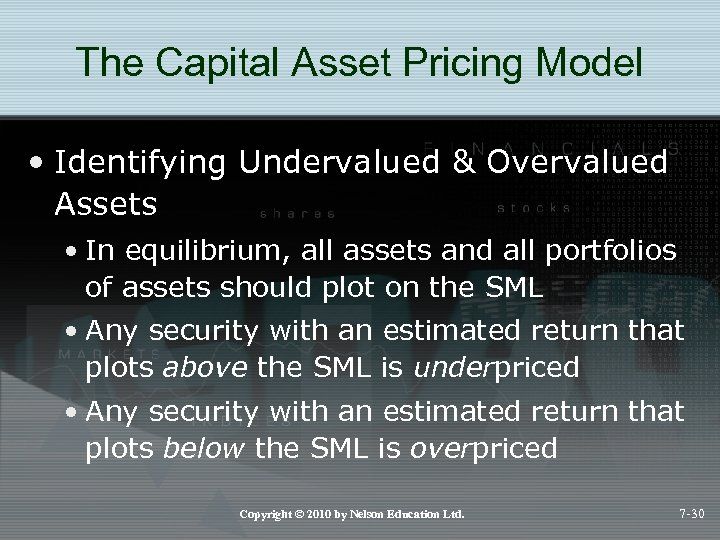The Capital Asset Pricing Model • Identifying Undervalued & Overvalued Assets • In equilibrium, all assets and all portfolios of assets should plot on the SML • Any security with an estimated return that plots above the SML is underpriced • Any security with an estimated return that plots below the SML is overpriced Copyright © 2010 by Nelson Education Ltd. 7 -30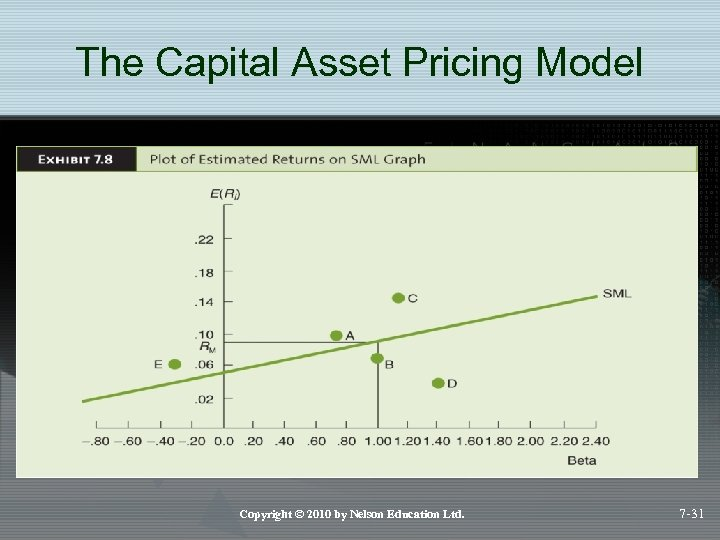The Capital Asset Pricing Model Copyright © 2010 by Nelson Education Ltd. 7 -31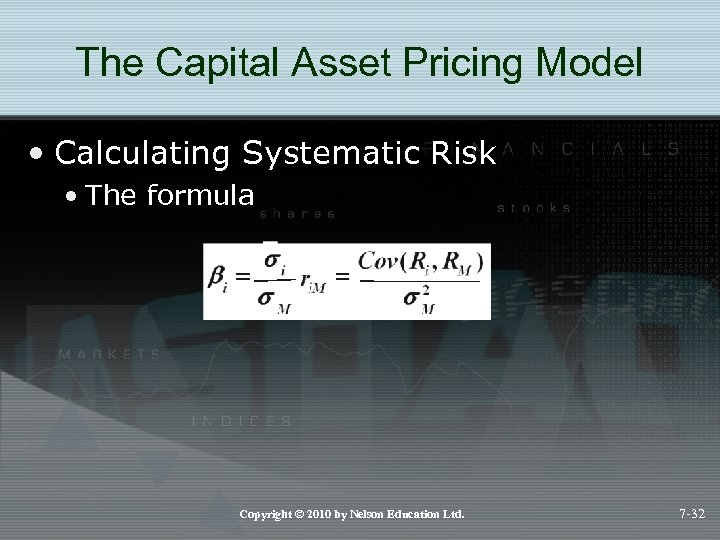The Capital Asset Pricing Model • Calculating Systematic Risk • The formula Copyright © 2010 by Nelson Education Ltd. 7 -32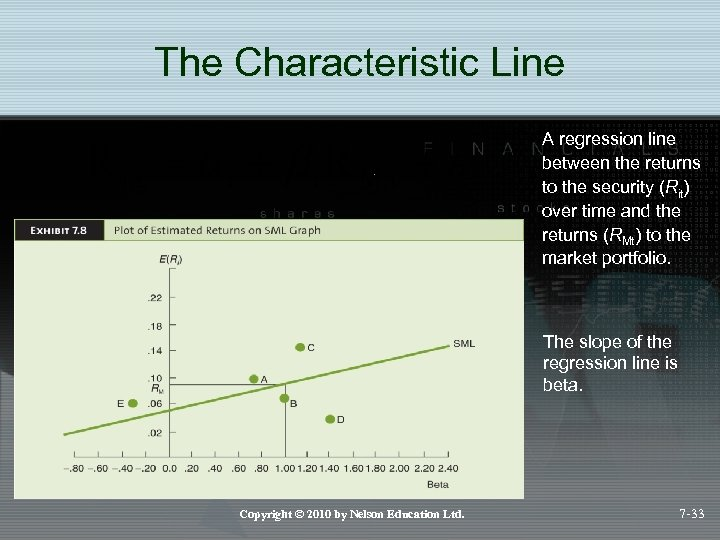The Characteristic Line A regression line between the returns to the security (Rit) over time and the returns (RMt) to the market portfolio. The slope of the regression line is beta. Copyright © 2010 by Nelson Education Ltd. 7 -33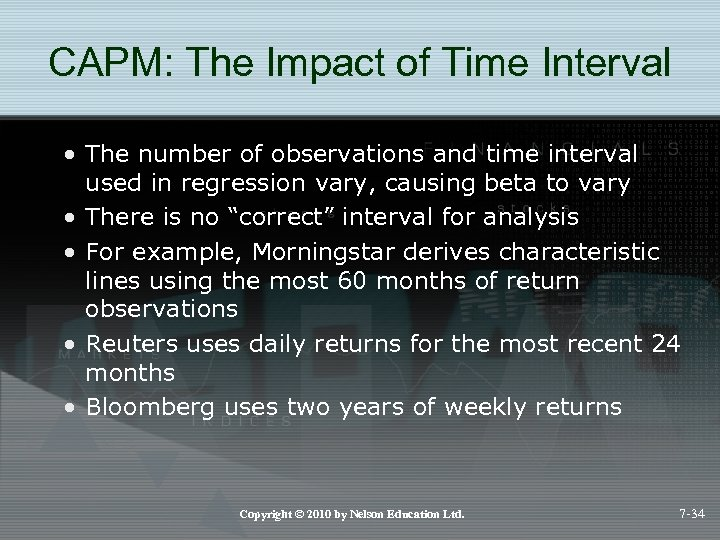CAPM: The Impact of Time Interval • The number of observations and time interval used in regression vary, causing beta to vary • There is no “correct” interval for analysis • For example, Morningstar derives characteristic lines using the most 60 months of return observations • Reuters uses daily returns for the most recent 24 months • Bloomberg uses two years of weekly returns Copyright © 2010 by Nelson Education Ltd. 7 -34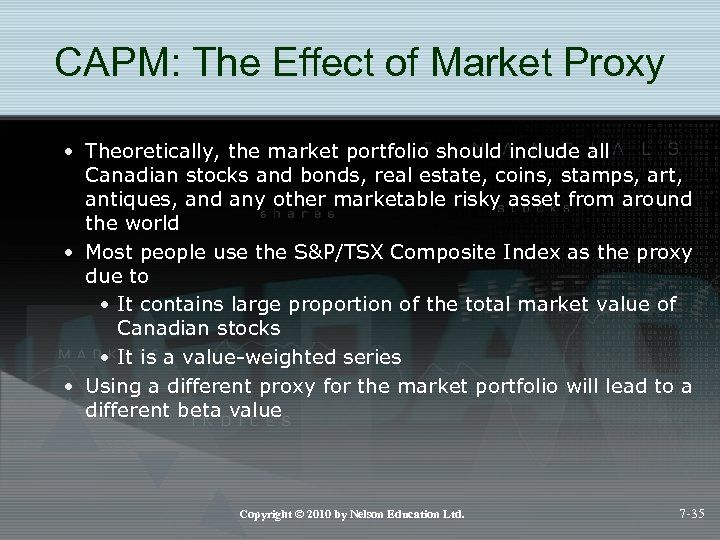CAPM: The Effect of Market Proxy • Theoretically, the market portfolio should include all Canadian stocks and bonds, real estate, coins, stamps, art, antiques, and any other marketable risky asset from around the world • Most people use the S&P/TSX Composite Index as the proxy due to • It contains large proportion of the total market value of Canadian stocks • It is a value-weighted series • Using a different proxy for the market portfolio will lead to a different beta value Copyright © 2010 by Nelson Education Ltd. 7 -35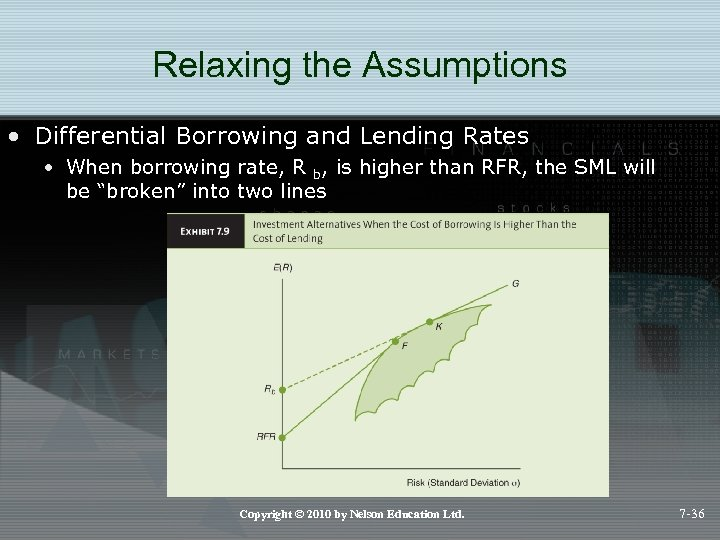Relaxing the Assumptions • Differential Borrowing and Lending Rates • When borrowing rate, R b, is higher than RFR, the SML will be “broken” into two lines Copyright © 2010 by Nelson Education Ltd. 7 -36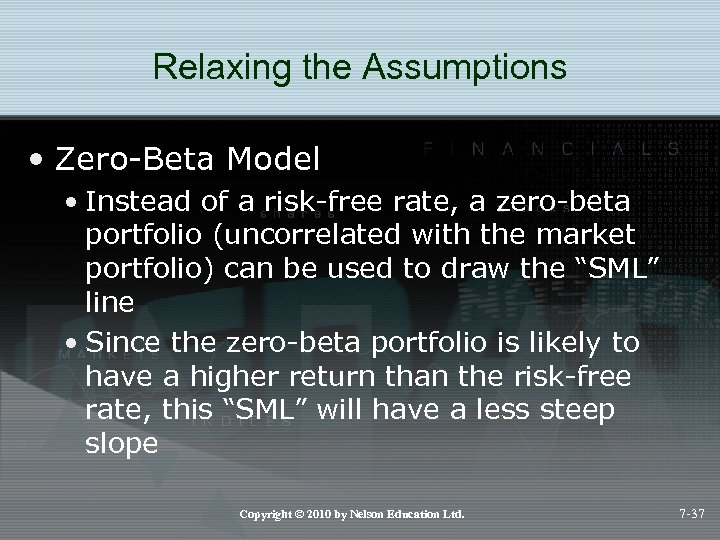Relaxing the Assumptions • Zero-Beta Model • Instead of a risk-free rate, a zero-beta portfolio (uncorrelated with the market portfolio) can be used to draw the “SML” line • Since the zero-beta portfolio is likely to have a higher return than the risk-free rate, this “SML” will have a less steep slope Copyright © 2010 by Nelson Education Ltd. 7 -37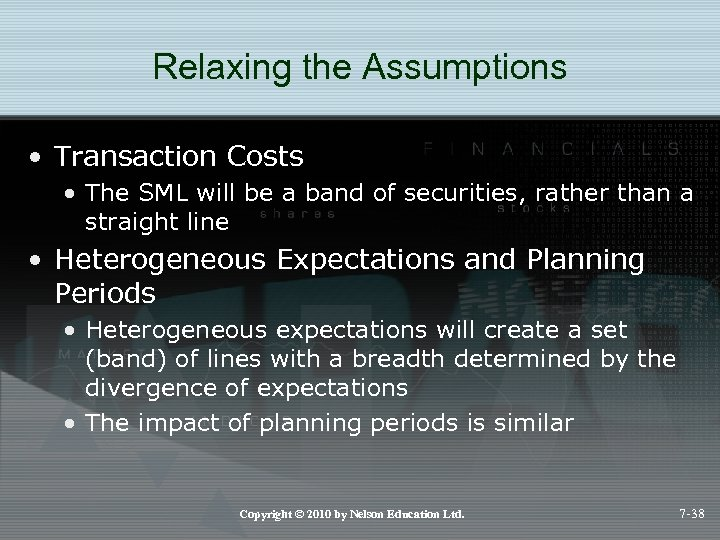Relaxing the Assumptions • Transaction Costs • The SML will be a band of securities, rather than a straight line • Heterogeneous Expectations and Planning Periods • Heterogeneous expectations will create a set (band) of lines with a breadth determined by the divergence of expectations • The impact of planning periods is similar Copyright © 2010 by Nelson Education Ltd. 7 -38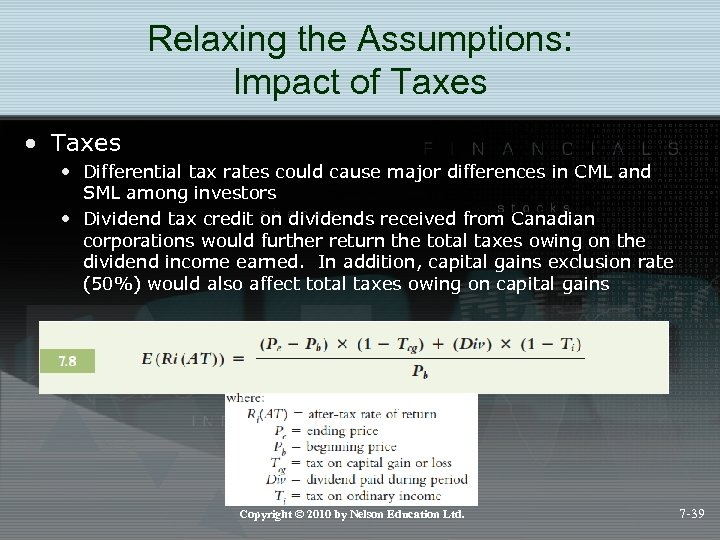Relaxing the Assumptions: Impact of Taxes • Differential tax rates could cause major differences in CML and SML among investors • Dividend tax credit on dividends received from Canadian corporations would further return the total taxes owing on the dividend income earned. In addition, capital gains exclusion rate (50%) would also affect total taxes owing on capital gains Copyright © 2010 by Nelson Education Ltd. 7 -39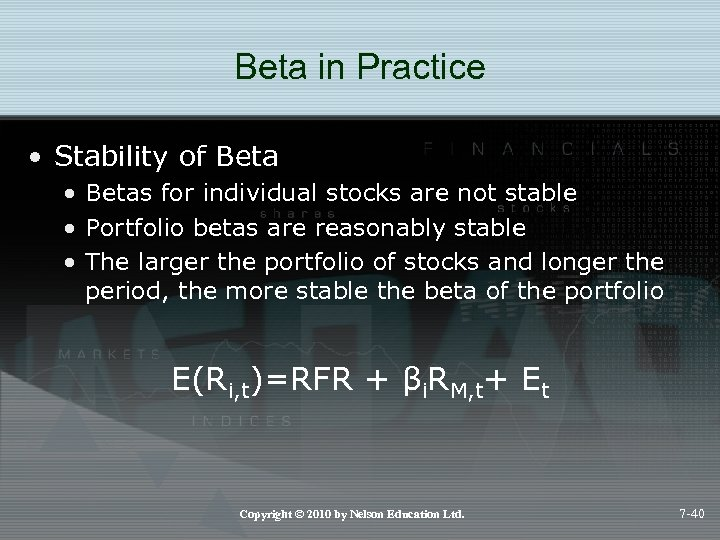Beta in Practice • Stability of Beta • Betas for individual stocks are not stable • Portfolio betas are reasonably stable • The larger the portfolio of stocks and longer the period, the more stable the beta of the portfolio E(Ri, t)=RFR + βi. RM, t+ Et Copyright © 2010 by Nelson Education Ltd. 7 -40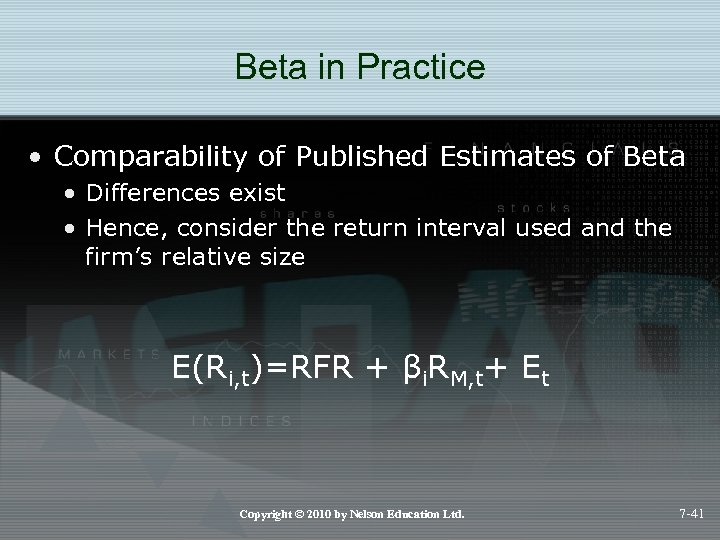Beta in Practice • Comparability of Published Estimates of Beta • Differences exist • Hence, consider the return interval used and the firm’s relative size E(Ri, t)=RFR + βi. RM, t+ Et Copyright © 2010 by Nelson Education Ltd. 7 -41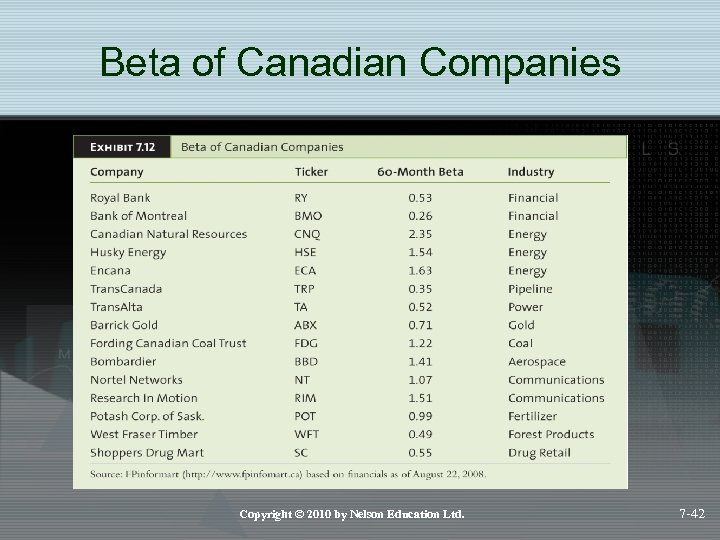Beta of Canadian Companies Copyright © 2010 by Nelson Education Ltd. 7 -42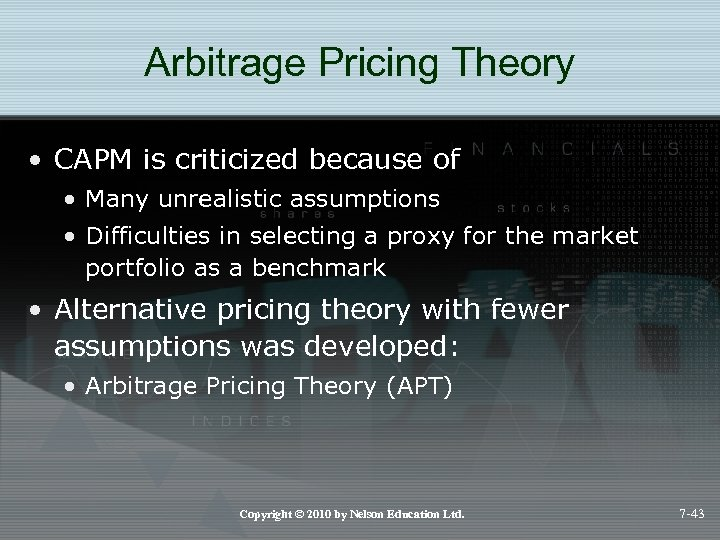Arbitrage Pricing Theory • CAPM is criticized because of • Many unrealistic assumptions • Difficulties in selecting a proxy for the market portfolio as a benchmark • Alternative pricing theory with fewer assumptions was developed: • Arbitrage Pricing Theory (APT) Copyright © 2010 by Nelson Education Ltd. 7 -43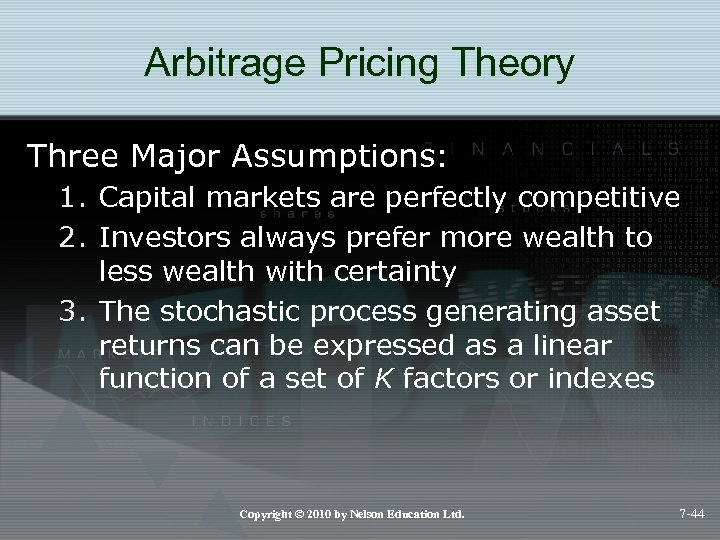Arbitrage Pricing Theory Three Major Assumptions: 1. Capital markets are perfectly competitive 2. Investors always prefer more wealth to less wealth with certainty 3. The stochastic process generating asset returns can be expressed as a linear function of a set of K factors or indexes Copyright © 2010 by Nelson Education Ltd. 7 -44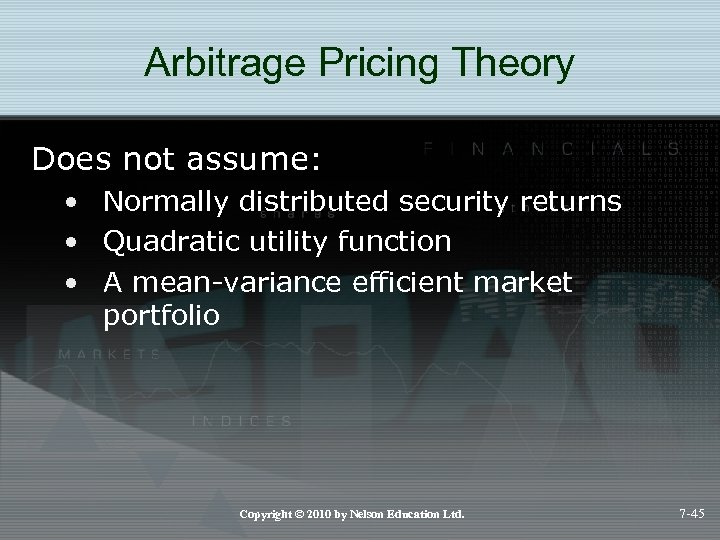Arbitrage Pricing Theory Does not assume: • Normally distributed security returns • Quadratic utility function • A mean-variance efficient market portfolio Copyright © 2010 by Nelson Education Ltd. 7 -45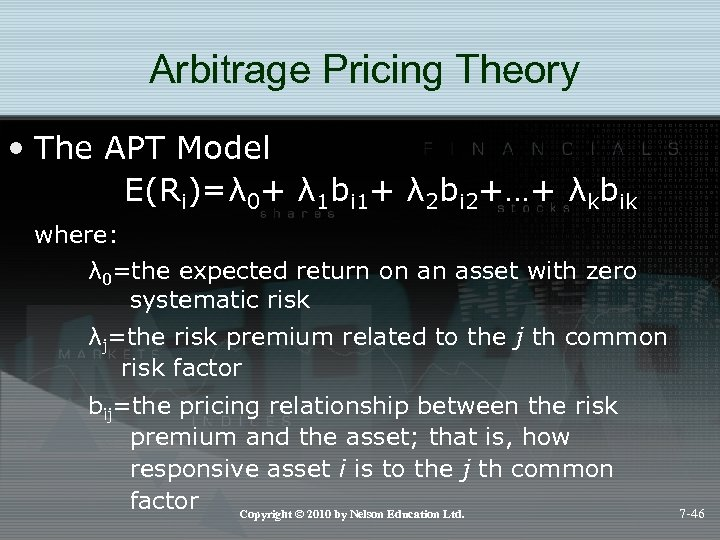Arbitrage Pricing Theory • The APT Model E(Ri)=λ 0+ λ 1 bi 1+ λ 2 bi 2+…+ λkbik where: λ 0=the expected return on an asset with zero systematic risk λj=the risk premium related to the j th common risk factor bij=the pricing relationship between the risk premium and the asset; that is, how responsive asset i is to the j th common factor Copyright © 2010 by Nelson Education Ltd. 7 -46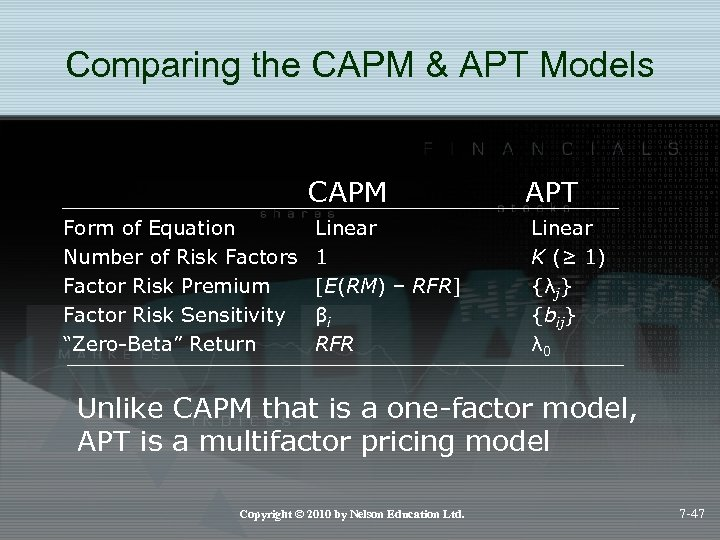Comparing the CAPM & APT Models CAPM Form of Equation Number of Risk Factors Factor Risk Premium Factor Risk Sensitivity “Zero-Beta” Return Linear 1 [E(RM) – RFR] βi RFR APT Linear K (≥ 1) {λj} {bij} λ 0 Unlike CAPM that is a one-factor model, APT is a multifactor pricing model Copyright © 2010 by Nelson Education Ltd. 7 -47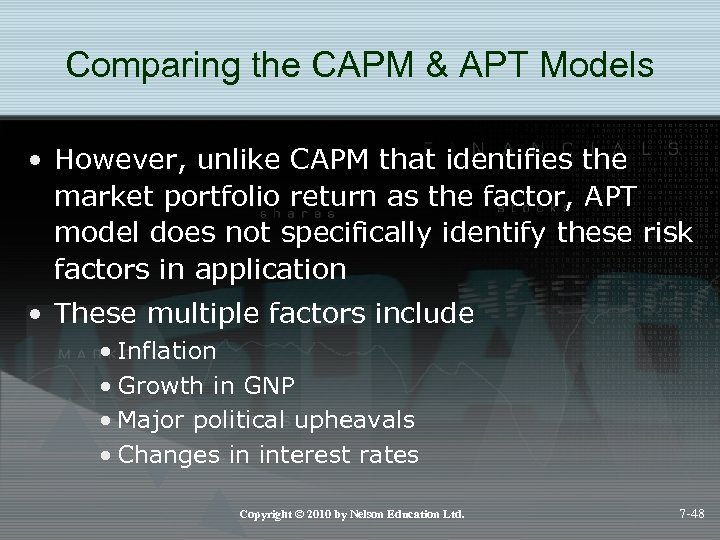Comparing the CAPM & APT Models • However, unlike CAPM that identifies the market portfolio return as the factor, APT model does not specifically identify these risk factors in application • These multiple factors include • Inflation • Growth in GNP • Major political upheavals • Changes in interest rates Copyright © 2010 by Nelson Education Ltd. 7 -48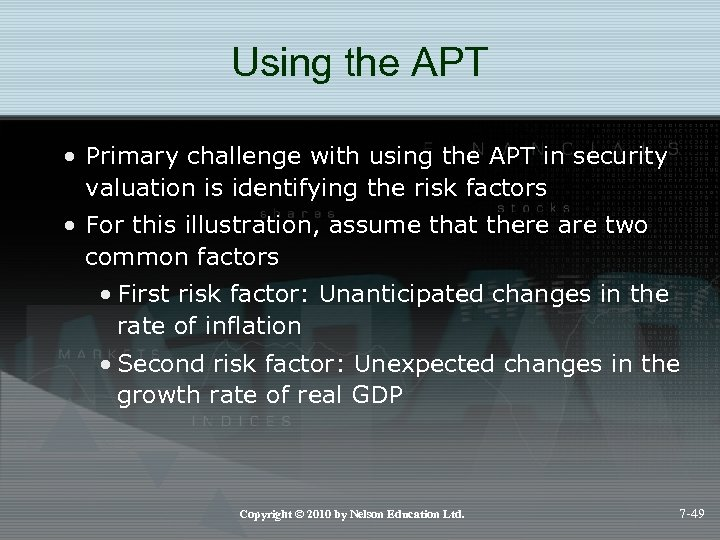Using the APT • Primary challenge with using the APT in security valuation is identifying the risk factors • For this illustration, assume that there are two common factors • First risk factor: Unanticipated changes in the rate of inflation • Second risk factor: Unexpected changes in the growth rate of real GDP Copyright © 2010 by Nelson Education Ltd. 7 -49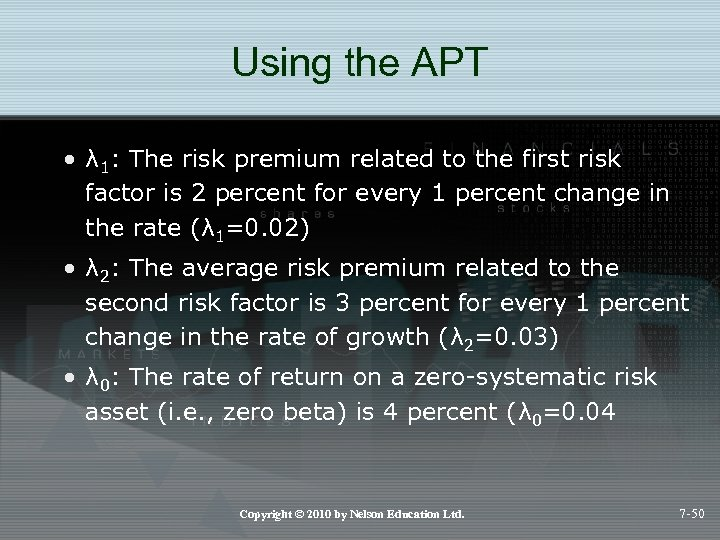Using the APT • λ 1: The risk premium related to the first risk factor is 2 percent for every 1 percent change in the rate (λ 1=0. 02) • λ 2: The average risk premium related to the second risk factor is 3 percent for every 1 percent change in the rate of growth (λ 2=0. 03) • λ 0: The rate of return on a zero-systematic risk asset (i. e. , zero beta) is 4 percent (λ 0=0. 04 Copyright © 2010 by Nelson Education Ltd. 7 -50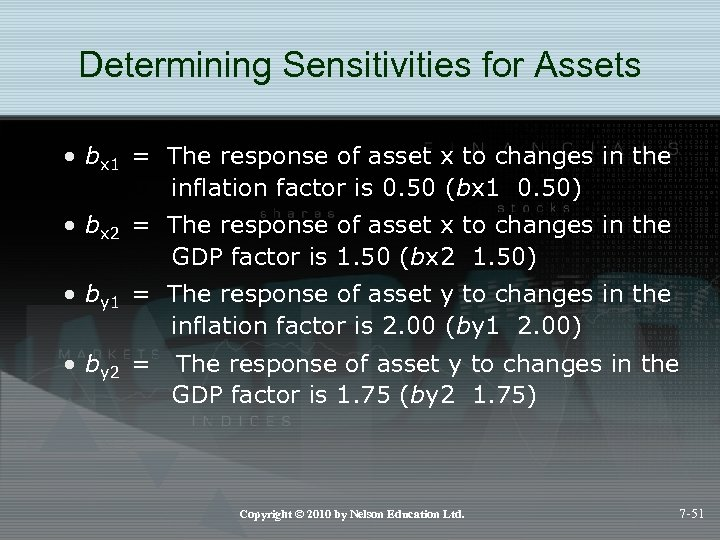Determining Sensitivities for Assets • bx 1 = The response of asset x to changes in the inflation factor is 0. 50 (bx 1 0. 50) • bx 2 = The response of asset x to changes in the GDP factor is 1. 50 (bx 2 1. 50) • by 1 = The response of asset y to changes in the inflation factor is 2. 00 (by 1 2. 00) • by 2 = The response of asset y to changes in the GDP factor is 1. 75 (by 2 1. 75) Copyright © 2010 by Nelson Education Ltd. 7 -51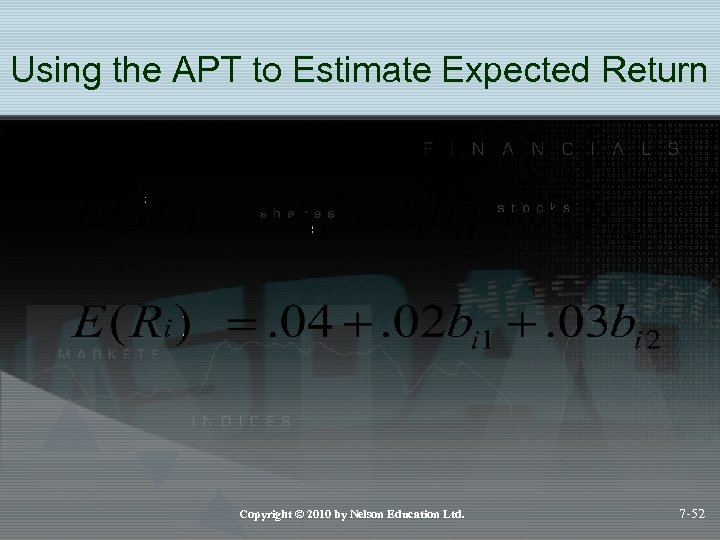Using the APT to Estimate Expected Return Copyright © 2010 by Nelson Education Ltd. 7 -52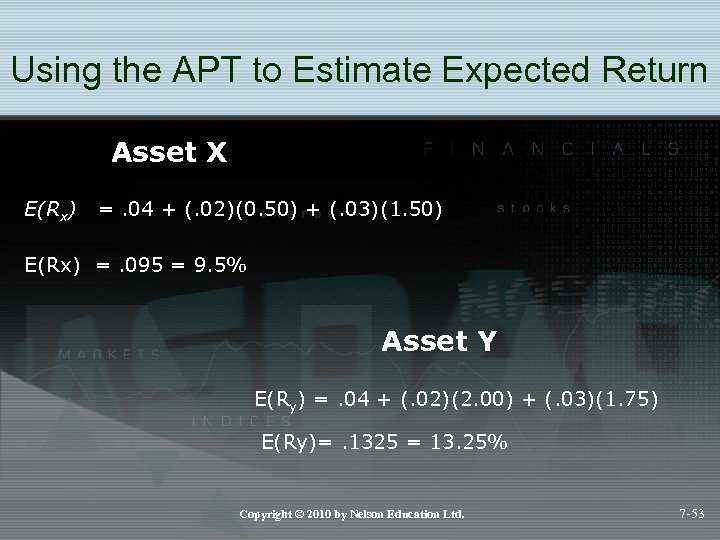Using the APT to Estimate Expected Return Asset X E(Rx) =. 04 + (. 02)(0. 50) + (. 03)(1. 50) E(Rx) =. 095 = 9. 5% Asset Y E(Ry) =. 04 + (. 02)(2. 00) + (. 03)(1. 75) E(Ry)=. 1325 = 13. 25% Copyright © 2010 by Nelson Education Ltd. 7 -53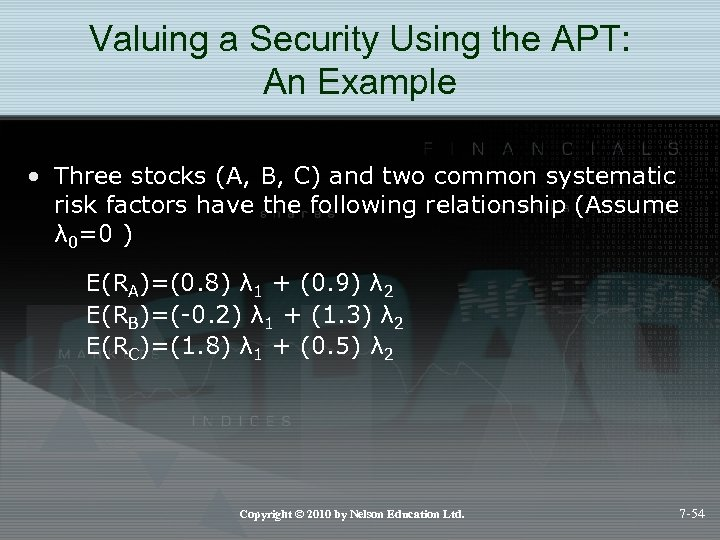Valuing a Security Using the APT: An Example • Three stocks (A, B, C) and two common systematic risk factors have the following relationship (Assume λ 0=0 ) E(RA)=(0. 8) λ 1 + (0. 9) λ 2 E(RB)=(-0. 2) λ 1 + (1. 3) λ 2 E(RC)=(1. 8) λ 1 + (0. 5) λ 2 Copyright © 2010 by Nelson Education Ltd. 7 -54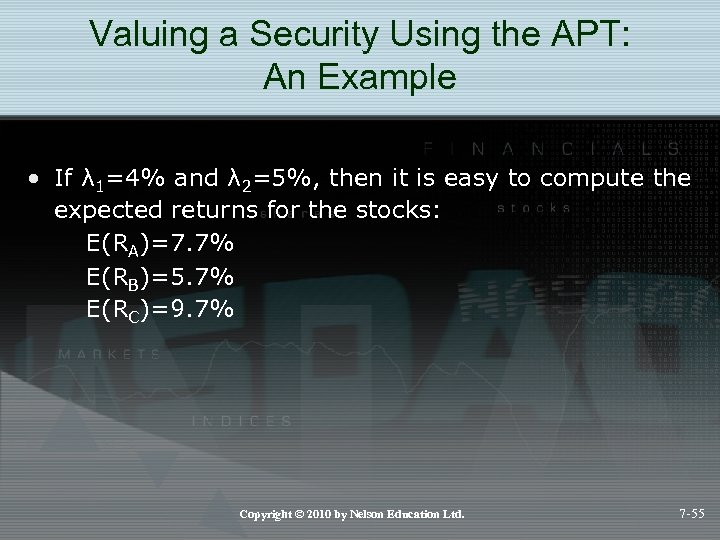Valuing a Security Using the APT: An Example • If λ 1=4% and λ 2=5%, then it is easy to compute the expected returns for the stocks: E(RA)=7. 7% E(RB)=5. 7% E(RC)=9. 7% Copyright © 2010 by Nelson Education Ltd. 7 -55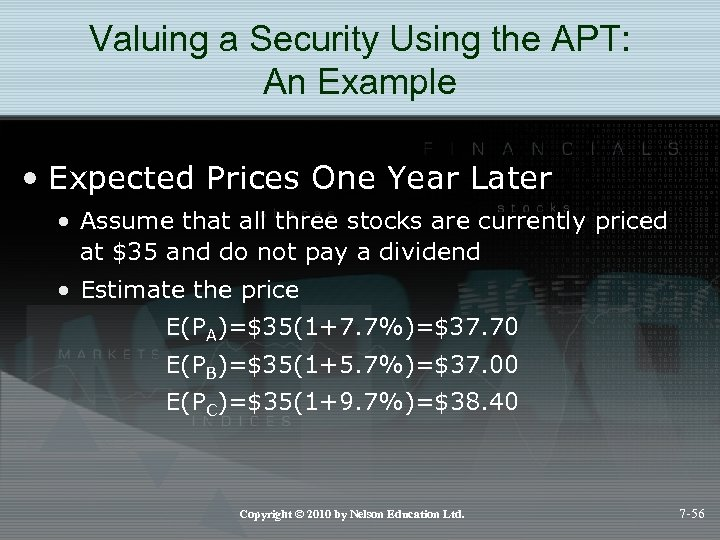Valuing a Security Using the APT: An Example • Expected Prices One Year Later • Assume that all three stocks are currently priced at \$35 and do not pay a dividend • Estimate the price E(PA)=\$35(1+7. 7%)=\$37. 70 E(PB)=\$35(1+5. 7%)=\$37. 00 E(PC)=\$35(1+9. 7%)=\$38. 40 Copyright © 2010 by Nelson Education Ltd. 7 -56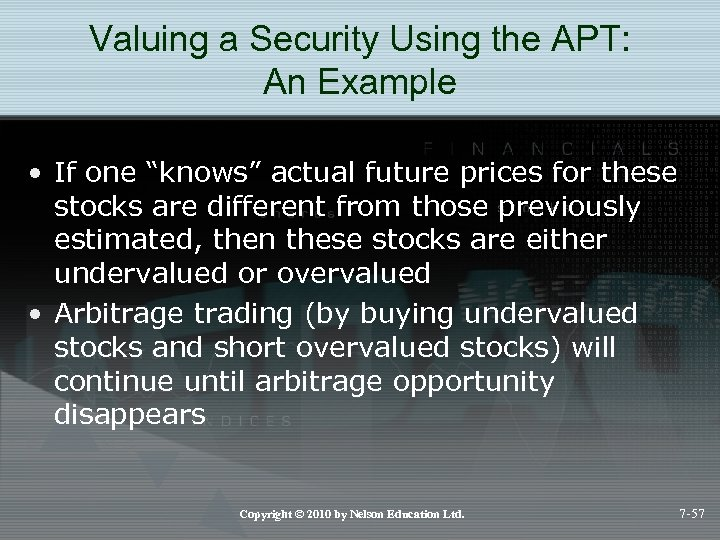Valuing a Security Using the APT: An Example • If one “knows” actual future prices for these stocks are different from those previously estimated, then these stocks are either undervalued or overvalued • Arbitrage trading (by buying undervalued stocks and short overvalued stocks) will continue until arbitrage opportunity disappears Copyright © 2010 by Nelson Education Ltd. 7 -57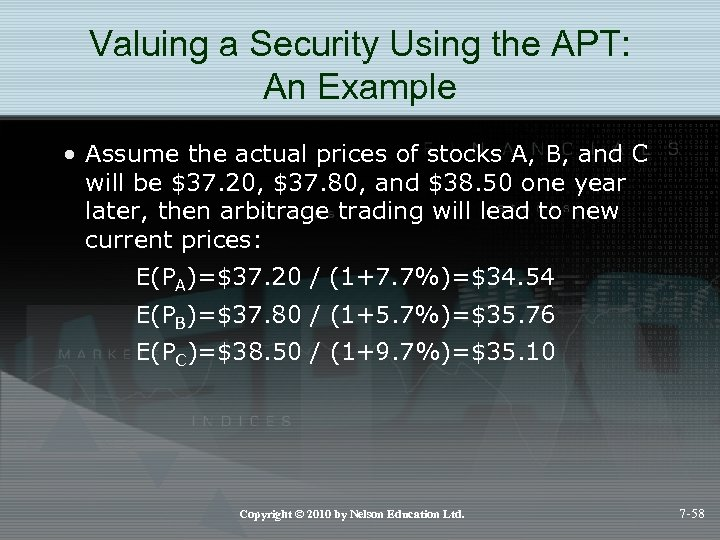Valuing a Security Using the APT: An Example • Assume the actual prices of stocks A, B, and C will be \$37. 20, \$37. 80, and \$38. 50 one year later, then arbitrage trading will lead to new current prices: E(PA)=\$37. 20 / (1+7. 7%)=\$34. 54 E(PB)=\$37. 80 / (1+5. 7%)=\$35. 76 E(PC)=\$38. 50 / (1+9. 7%)=\$35. 10 Copyright © 2010 by Nelson Education Ltd. 7 -58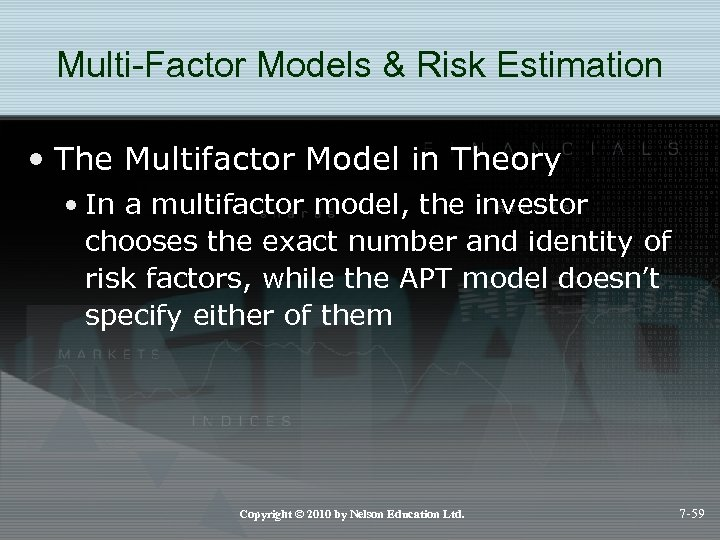Multi-Factor Models & Risk Estimation • The Multifactor Model in Theory • In a multifactor model, the investor chooses the exact number and identity of risk factors, while the APT model doesn’t specify either of them Copyright © 2010 by Nelson Education Ltd. 7 -59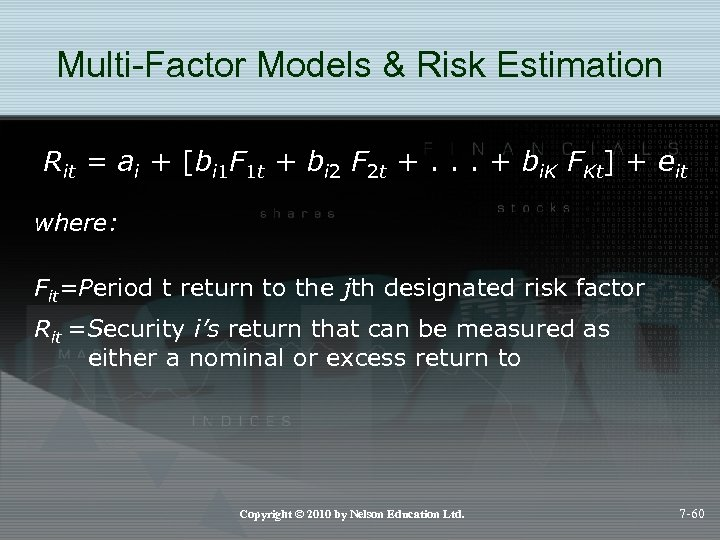Multi-Factor Models & Risk Estimation Rit = ai + [bi 1 F 1 t + bi 2 F 2 t +. . . + bi. K FKt] + eit where: Fit=Period t return to the jth designated risk factor Rit =Security i’s return that can be measured as either a nominal or excess return to Copyright © 2010 by Nelson Education Ltd. 7 -60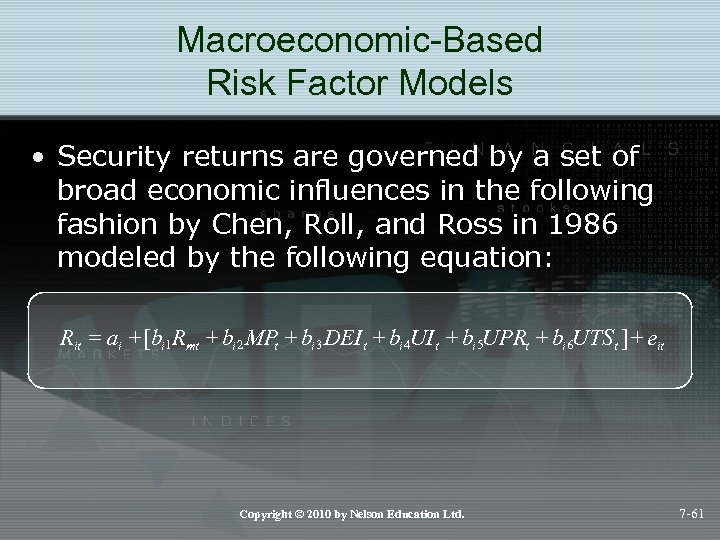Macroeconomic-Based Risk Factor Models • Security returns are governed by a set of broad economic influences in the following fashion by Chen, Roll, and Ross in 1986 modeled by the following equation: Rit = ai + [bi 1 Rmt + bi 2 MPt + bi 3 DEI t + bi 4 UI t + bi 5 UPRt + bi 6 UTSt ] + eit Copyright © 2010 by Nelson Education Ltd. 7 -61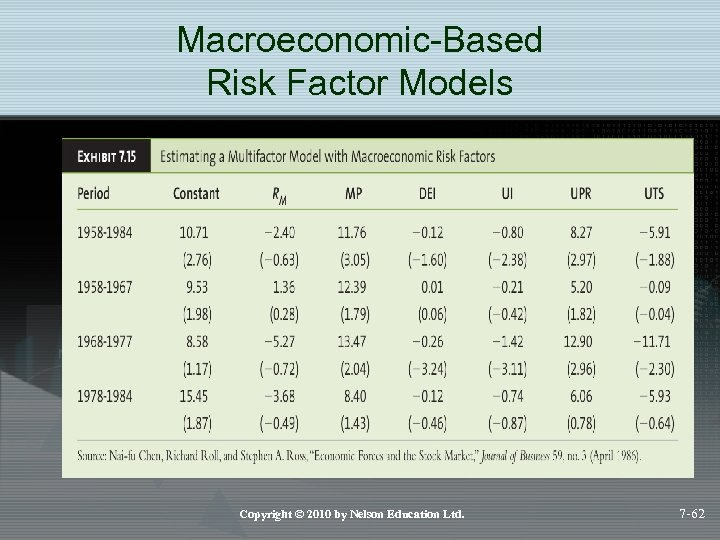Macroeconomic-Based Risk Factor Models Copyright © 2010 by Nelson Education Ltd. 7 -62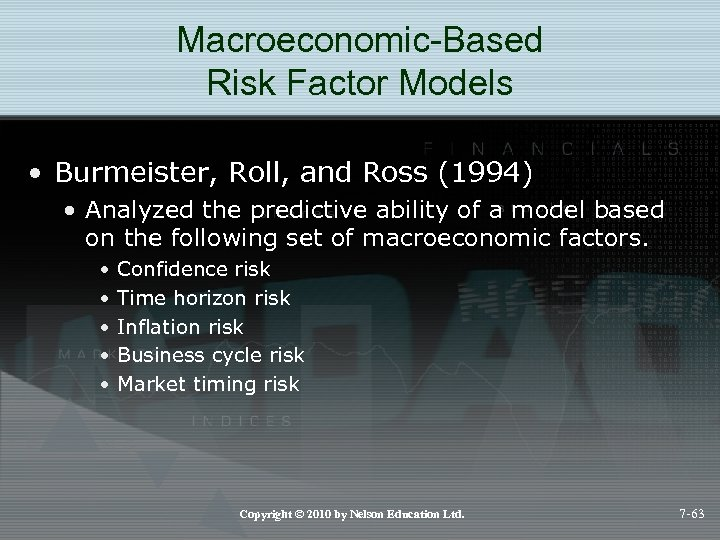Macroeconomic-Based Risk Factor Models • Burmeister, Roll, and Ross (1994) • Analyzed the predictive ability of a model based on the following set of macroeconomic factors. • • • Confidence risk Time horizon risk Inflation risk Business cycle risk Market timing risk Copyright © 2010 by Nelson Education Ltd. 7 -63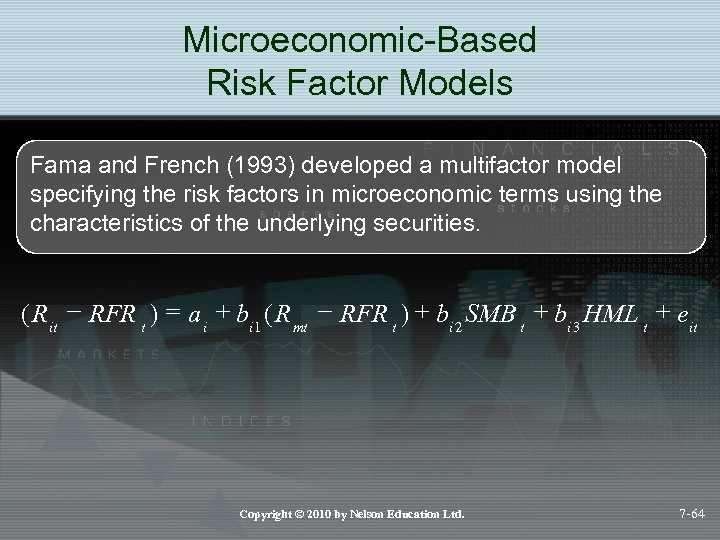Microeconomic-Based Risk Factor Models Fama and French (1993) developed a multifactor model specifying the risk factors in microeconomic terms using the characteristics of the underlying securities. ( Rit - RFR t ) = a i + bi 1 ( R mt - RFR t ) + bi 2 SMB t + bi 3 HML t + eit Copyright © 2010 by Nelson Education Ltd. 7 -64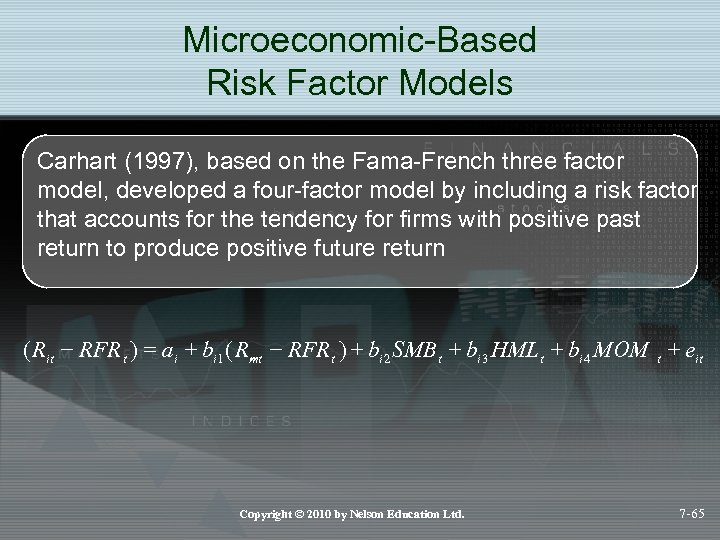Microeconomic-Based Risk Factor Models Carhart (1997), based on the Fama-French three factor model, developed a four-factor model by including a risk factor that accounts for the tendency for firms with positive past return to produce positive future return ( Rit - RFR t ) = ai + bi 1 ( Rmt - RFR t ) + bi 2 SMB t + bi 3 HML t + bi 4 MOM t + eit Copyright © 2010 by Nelson Education Ltd. 7 -65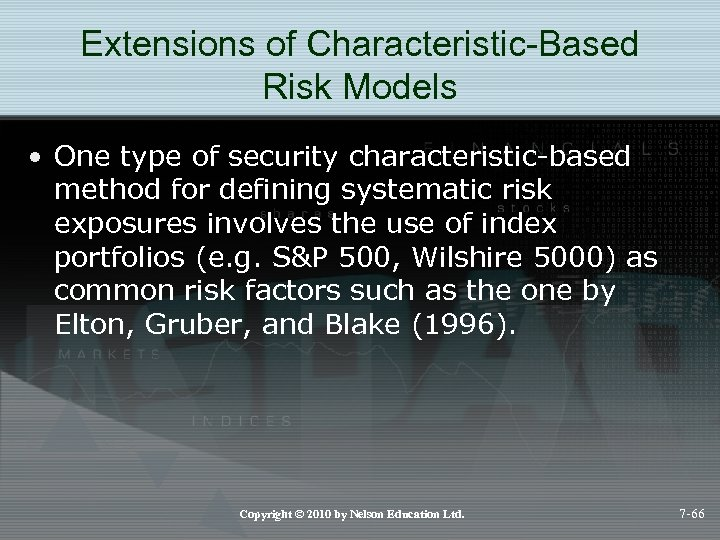Extensions of Characteristic-Based Risk Models • One type of security characteristic-based method for defining systematic risk exposures involves the use of index portfolios (e. g. S&P 500, Wilshire 5000) as common risk factors such as the one by Elton, Gruber, and Blake (1996). Copyright © 2010 by Nelson Education Ltd. 7 -66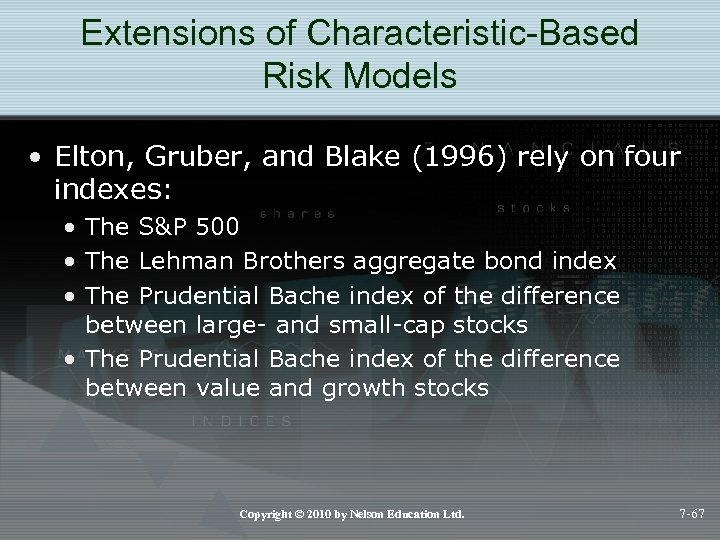Extensions of Characteristic-Based Risk Models • Elton, Gruber, and Blake (1996) rely on four indexes: • The S&P 500 • The Lehman Brothers aggregate bond index • The Prudential Bache index of the difference between large- and small-cap stocks • The Prudential Bache index of the difference between value and growth stocks Copyright © 2010 by Nelson Education Ltd. 7 -67